GeeksforGeeks App
Open AppBrowser
Continue

# ML | Boston Housing Kaggle Challenge with Linear Regression

Boston Housing Data: This dataset was taken from the StatLib library and is maintained by Carnegie Mellon University. This dataset concerns the housing prices in the housing city of Boston. The dataset provided has 506 instances with 13 features.
The Description of the dataset is taken from the below reference as shown in the table follows: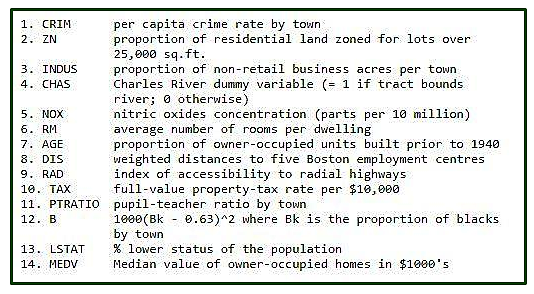Let’s make the Linear Regression Model, predicting housing prices by Inputting Libraries and datasets.

## Python3

 `# Importing Libraries``import` `numpy as np``import` `pandas as pd``import` `matplotlib.pyplot as plt`` ` `# Importing Data``from` `sklearn.datasets ``import` `load_boston``boston ``=` `load_boston()`

The shape of input Boston data and getting feature_names.

## Python3

 `boston.data.shape`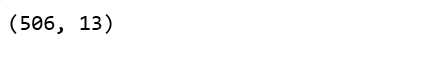## Python3

 `boston.feature_names`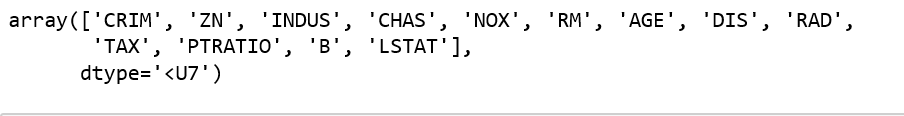Converting data from nd-array to data frame and adding feature names to the data

## Python3

 `data ``=` `pd.DataFrame(boston.data)``data.columns ``=` `boston.feature_names` `data.head(``10``)`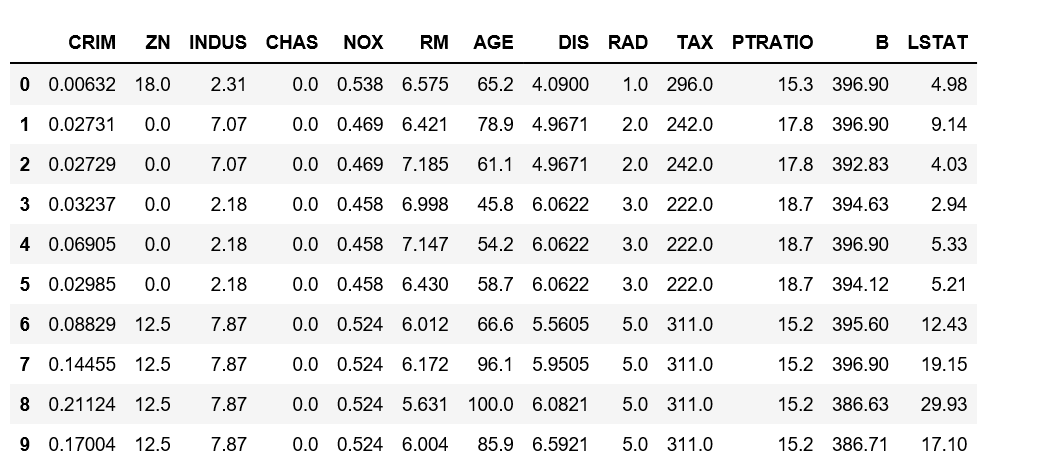Adding the ‘Price’ column to the dataset

## Python3

 `# Adding 'Price' (target) column to the data``boston.target.shape`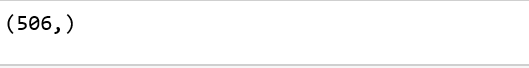## Python3

 `data[``'Price'``] ``=` `boston.target``data.head()`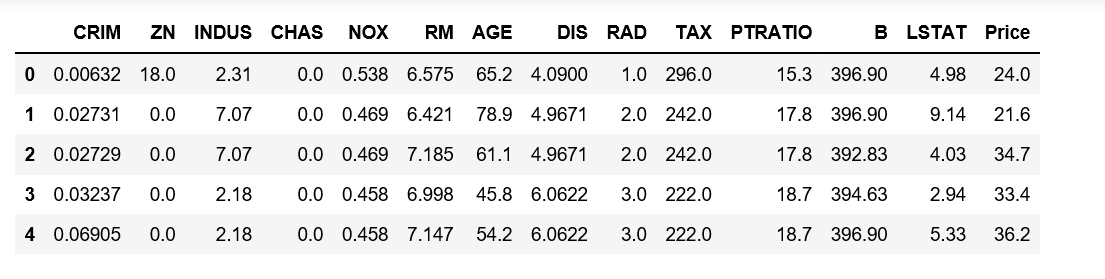Description of Boston dataset

## Python3

 `data.describe()`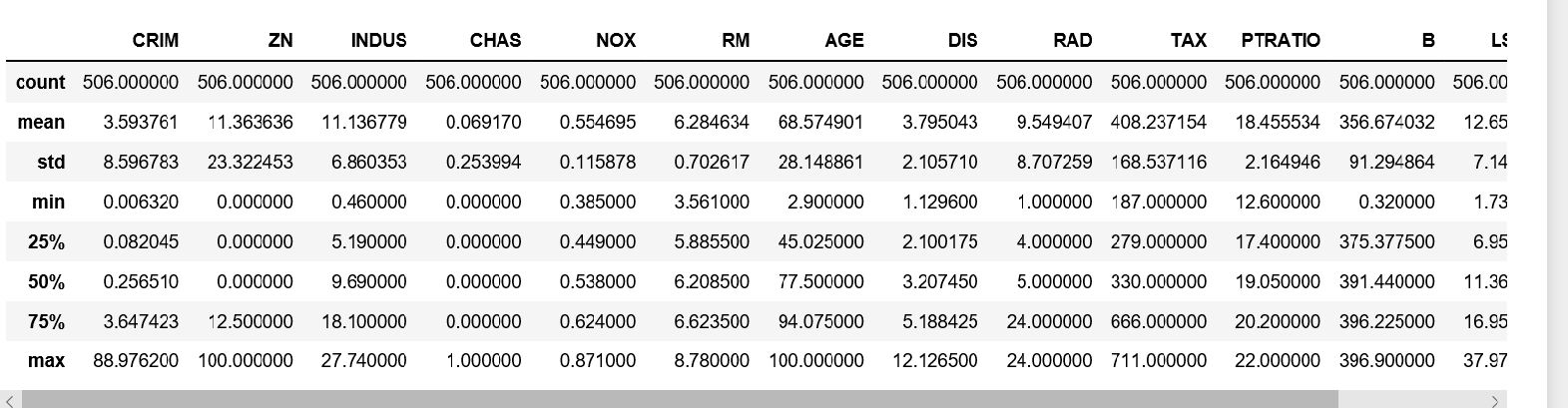Info of Boston Dataset

## Python3

 `data.info()`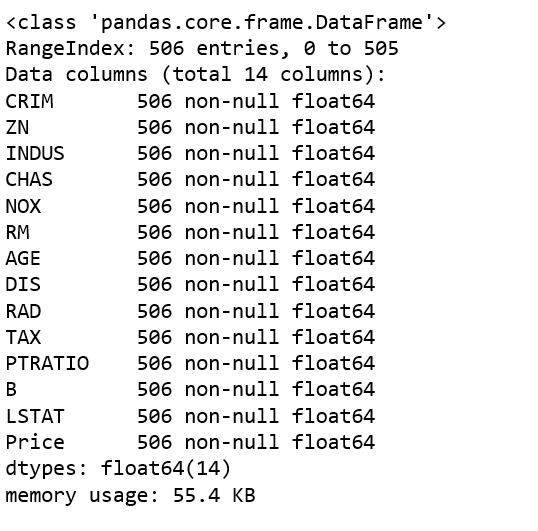Getting input and output data and further splitting data to training and testing dataset.

## Python3

 `# Input Data``x ``=` `boston.data`` ` `# Output Data``y ``=` `boston.target`` ` ` ` `# splitting data to training and testing dataset.` `#from sklearn.cross_validation import train_test_split``#the submodule cross_validation is renamed and deprecated to model_selection``from` `sklearn.model_selection ``import` `train_test_split` `xtrain, xtest, ytrain, ytest ``=` `train_test_split(x, y, test_size ``=``0.2``,``                                                    ``random_state ``=` `0``)`` ` `print``(``"xtrain shape : "``, xtrain.shape)``print``(``"xtest shape  : "``, xtest.shape)``print``(``"ytrain shape : "``, ytrain.shape)``print``(``"ytest shape  : "``, ytest.shape)`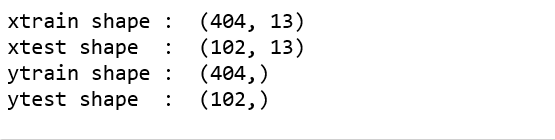Applying Linear Regression Model to the dataset and predicting the prices.

## Python3

 `# Fitting Multi Linear regression model to training model``from` `sklearn.linear_model ``import` `LinearRegression``regressor ``=` `LinearRegression()``regressor.fit(xtrain, ytrain)`` ` `# predicting the test set results``y_pred ``=` `regressor.predict(xtest)`

Plotting Scatter graph to show the prediction results – ‘y_true’ value vs ‘y_pred’ value.

## Python3

 `# Plotting Scatter graph to show the prediction``# results - 'ytrue' value vs 'y_pred' value``plt.scatter(ytest, y_pred, c ``=` `'green'``)``plt.xlabel(``"Price: in \$1000's"``)``plt.ylabel(``"Predicted value"``)``plt.title(``"True value vs predicted value : Linear Regression"``)``plt.show()`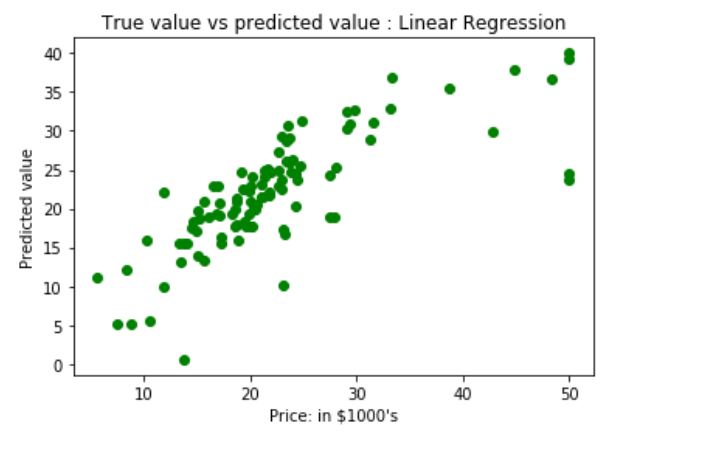Results of Linear Regression i.e. Mean Squared Error and Mean Absolute Error.

## Python3

 `from` `sklearn.metrics ``import` `mean_squared_error, mean_absolute_error``mse ``=` `mean_squared_error(ytest, y_pred)``mae ``=` `mean_absolute_error(ytest,y_pred)``print``(``"Mean Square Error : "``, mse)``print``(``"Mean Absolute Error : "``, mae)`

```Mean Square Error :  33.448979997676496
Mean Absolute Error :  3.8429092204444966```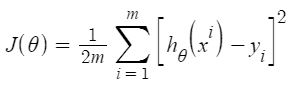As per the result, our model is only 66.55% accurate. So, the prepared model is not very good for predicting housing prices. One can improve the prediction results using many other possible machine learning algorithms and techniques.

Here are a few further steps on how you can improve your model.

My Personal Notes arrow_drop_up